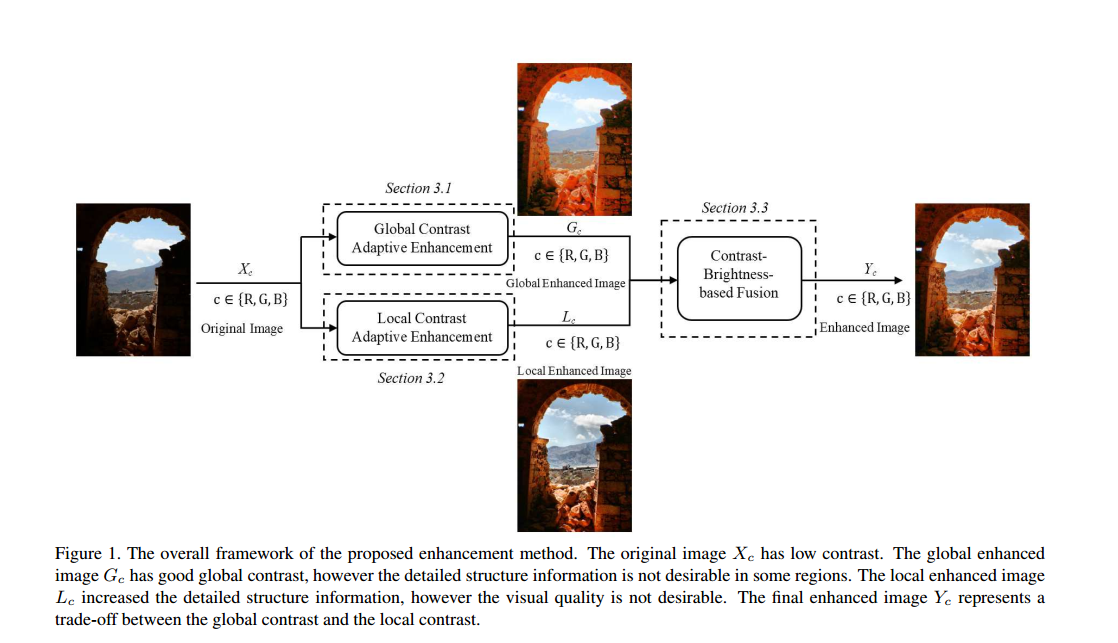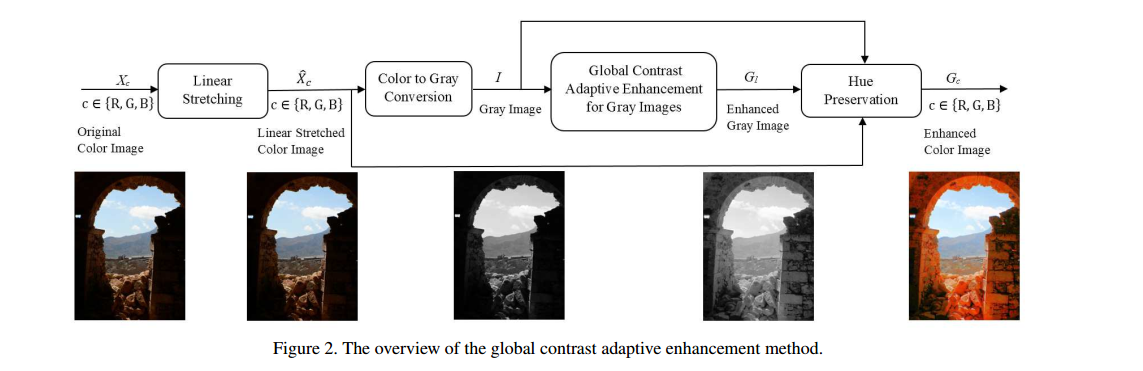This paper comes from the 2017 ICCV,which introduce a method for image enhancement that is not related to deep learning.Here I summarize this paper’s content as follows:

#### The main idea

The main idea of this article is that it introduce a method for Non-uniform Illumination image enhancement.First,it use a global contrast adaptive enhancement algorithm to obtain the global enhancement image,then it use a hue preserving local contrast adaptive enhancement algorithm to produce local enhancement image,finally a contast-brightness fusion algorithm fuse the two parts,which represent a trade-off between the global contrast and local contrast.

In the previous,the enhancement can atribute to two main methods which is histogram-based methods and filter-based methods repectively……

#### Main work

The Over view of contrast adaptive enhancement method is illustrated as follows:##### Global Contrast Adaptive Enhancement

The global contrast adaptive enhancement method is shwns as follows:The motivation of this step is that many image enhancement methods treat the three color channels respectively,which will change the hue of original images,so it is very important to preserve the hue component in images.Note that the two images with the values (r1,g1,b1) and (r2,g2,b2) have the same hue if the equation (r1,g1,b1)=a(r2,g2,b2)+d(1,1,1) exist.First,the value range of the input image $X_c$ is converted to the full range[0,L-1] with linear stretching:

where the $X{min}$and$X{max}$ are respectively the minimal and the maximal intensity values among the three color channels of the image $X_c$,and L=256 for 8 bit images.
The stretched image is converted to the corresponding intensity image I using the follow equation:

Then a novel contrast enhancement algorithm is applied to I to obtain the corresponding enhanced intensity image $G_I$,this method will be introduced later.Finally,it use the hue preservation enhancement framework to obtain the final enhanced color image $G_c$ with:

Here it is necessary to inroduce the Global Contrast Adaptive Enhancement for Gray Images.Usually the historam-based method obtains the corresponding mapping function to modify the pixel values and enhancing the contast of the considered image.The mapping function T can be shown as follows:

The problem of this mapping function may generate unnatural looking images.Since the article proposed a new method which means that the modified histogram h should be closer to the normalized uniformed historgram $h_U$ and the value of the residual $h-h_I$ should be small,too.Thus the problem of getting the optimal histogram $\hat{h}$can be shown as follows:

where the $\lambda$ can adjusts the trade-off between the contrast enhancement and the data fidelity.By transfering it to L2 norm,the solution can be showns as follows:

It should be noticed that the arameter λ needs to be carefully tuned for obtaining satisfied enhancement results.Since the goal is to adaptive enhance the contrast of the considered image,this article adopt the tone distortion of the mapping function T to guide the optimization,which can be shown as follows:

In this definition, we can know that the smaller the tone distortion D(T) the smoother the tone reproduced by the mapping function T.The smoother tone means less unnatural looking in the enhanced image.This mapping function can be utilized to adaptively enhance images.

##### Local Contrast Adaptive Enhancement

It is also necessary to enhance the local contrast of the images.First it use the hue preservation enhancement framework in the (3),then it use the Contrast-Limited
Adaptive Histogram Equalization method to improve the local contrast and preserve the hue of the considered image.The framework is similar to Global Contrast Adaptive Enhancement.

##### Contrast-Brightness-based Fusion

Finally it should combine the globally enhanced image $G_c$ and locally enhanced image $L_c$ to get finall output.This fusion framework adopt contrast-brightness-based pixel-level weights to fuse every pixel,which can be showns as follows:

where $W_d$ is the weight map, $C_d$ is the contrast measure, $B_d$ is the brightness measure, and the operation min can efficiently penalize the corresponding low-contrast, low brightness (under-exposure) or high brightness (over-exposure).$C_d$ is obtained by a Laplacian filter, which can assign high weights to edges and textures in the corresponding image,$B_d$ is computed by a Gaussian curve,which can assign high weights to pixel values close to 0.5 and define low weights to pixel values near 0 (under-exposure) or near 1 (over-exposure).Thus,the weight map can be shown as follows:

Finally,the fusion result is:

Note the results computed directly usually contain the undesired seam problem in the
fused image, so it adopt a Laplacian pyramid method to avoid this problem.

#### Experiments

…….

Translation help:
stretching:拉伸
seam:接缝

0%